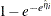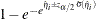#### Cumulative Response Models

For a row vector of explanatory variables, the linear predictor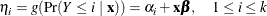is estimated by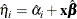where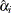and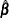are the MLEs of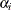and. The estimated standard error of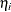is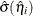, which can be computed as the square root of the quadratic form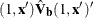, where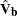is the estimated covariance matrix of the parameter estimates. The asymptotic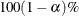confidence interval foris given by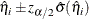where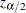is the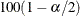percentile point of a standard normal distribution.

The predicted value and theconfidence limits for Pr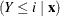are obtained by back-transforming the corresponding measures for the linear predictor.

Predicted ProbabilityConfidence Limits

LOGIT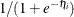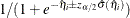PROBIT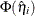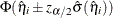CLOGLOG